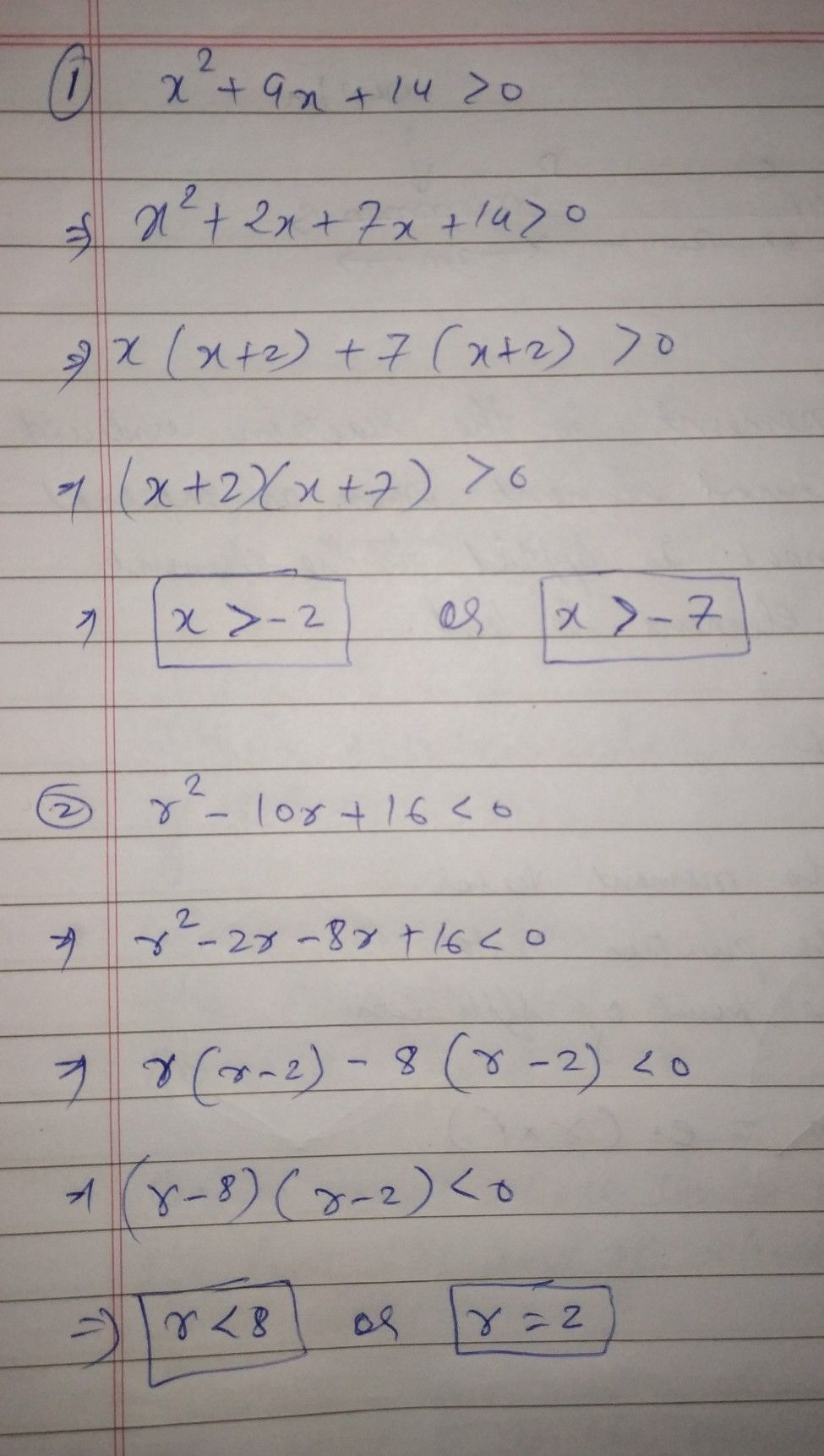Symbol
ProblemFind the solution set of each of the following quadratic inequalities then graph. Answer the questions that follow. $1$ $x^{2}+9x+14>0$ $2$ $r^{2}-10r+16<0$ $3$ $x^{2}+6x\geq -5$ $4$ $m^{2}-7m\leq 10$ $5.$ $x^{2}-5x-14>0$
7th-9th grade
Algebra
Search count: 139
SolutionQanda teacher - rakhi singplease ask one question at a timeStudent
is that the solution ?
did you read the directions?Qanda teacher - rakhi sing
yes
it is the right solutionStudent
ok sir thanks a lot.